# Python 基础（二） | Python 的基本数据类型

• 2022 年 9 月 08 日
山东
• 本文字数：4240 字

阅读完需：约 14 分钟

# 第二章 基本数据类型

⭐本专栏旨在对 Python 的基础语法进行详解，精炼地总结语法中的重点，详解难点，面向零基础及入门的学习者，通过专栏的学习可以熟练掌握 python 编程，同时为后续的数据分析，机器学习及深度学习的代码能力打下坚实的基础。

🔥本文已收录于 Python 基础系列专栏： Python基础系列教程 欢迎订阅，持续更新。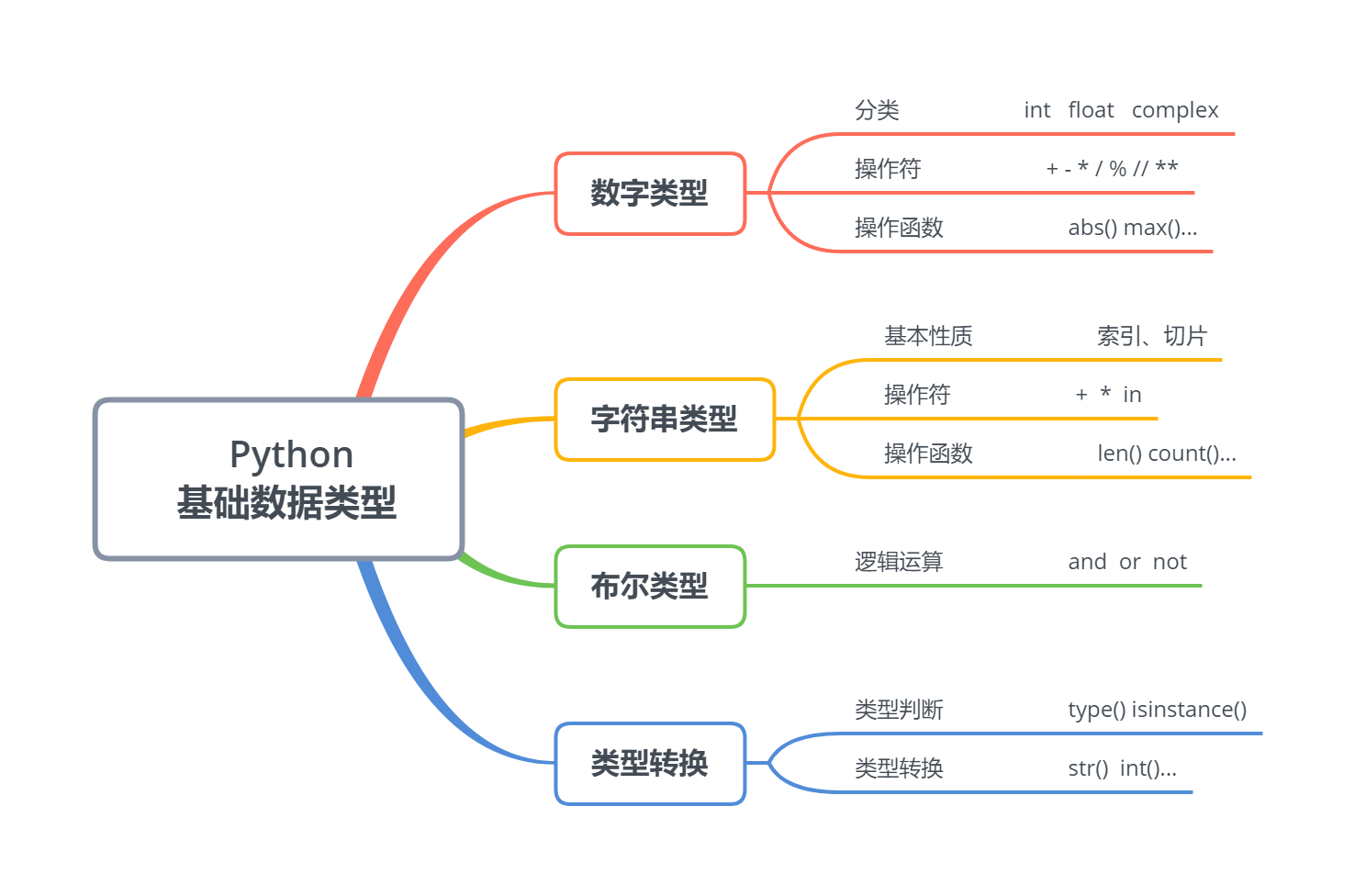# 第一部分 数字类型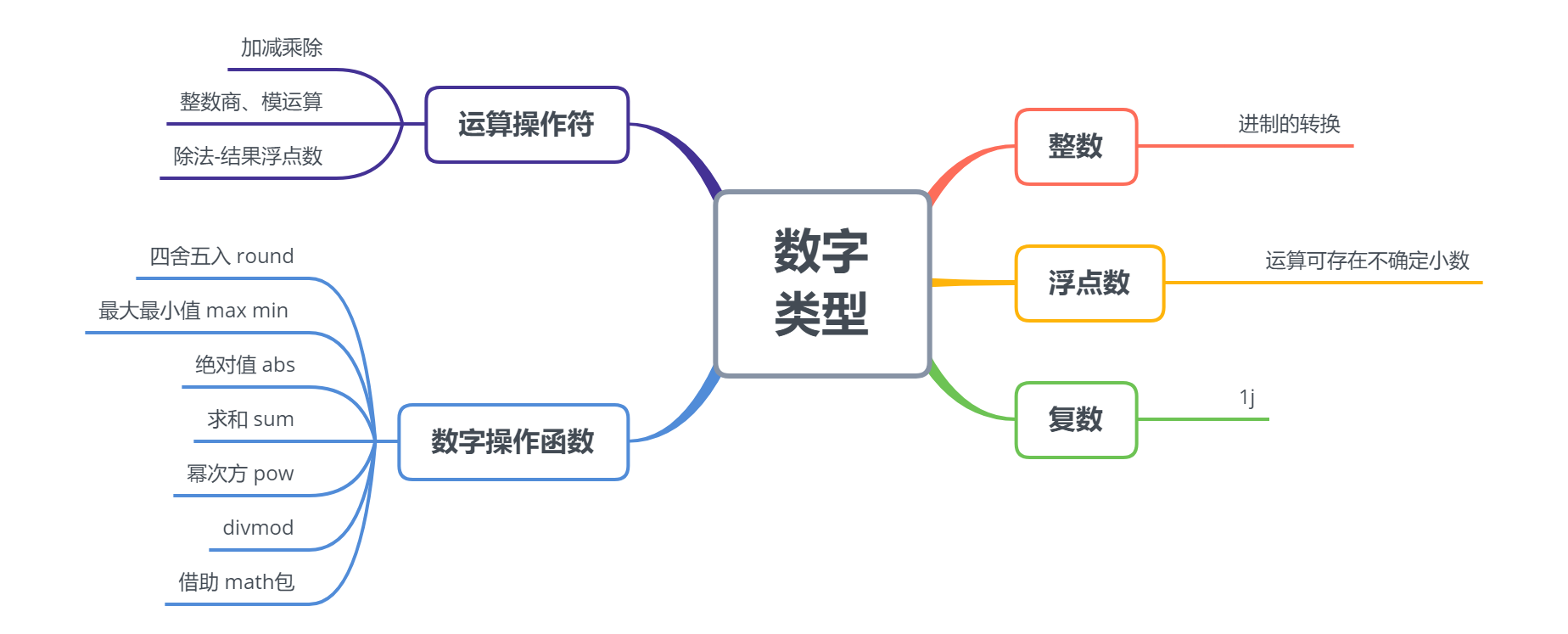## 1.1 数字类型的组成

### 1.1.1 整数——不同进制的转换

• 默认输入十进制

• 二进制 0b、八进制 0o、十六进制 0x

16 == 0b10000 == 0o20 == 0x10

True

• 十进制与其他进制的转换

a = bin(16)   # 转二进制b = oct(16)   # 转八进制c = hex(16)   # 转十六进制print(a, b, c)

0b10000 0o20 0x10

a == b == c

False

type(a)

str

• 其他进制转十进制

d = int(a, 2)      # 二进制转十进制e = int(b, 8)      # 八进制转十进制f = int(c, 16)     # 十六进制转十进制print(d, e, f)

16 16 16

### 1.1.2 浮点数——不确定性

• 不确定小数问题

(0.1+0.2) == 0.3

False

0.1+0.2

0.30000000000000004

• 原因：部分小数不能用二进制小数完全表示

• 0.00011001100110011001 0.099999427795410160.0011001100110011 0.19999694824218750.01001100110011001 0.299995422363281250.01100110011001101 0.400001525878906250.1 === === 0.5

• 通常情况下不会影响计算精度

0.1 + 0.7

0.7999999999999999

• 四舍五入获得精确解

• 可以采用四舍五入的方式解决：round(parameter, 保留小数位数)

a = 3*0.1print(a)

0.30000000000000004

b = round(a, 1)print(b)b == 0.3

0.3True

### 1.1.3 复数——a+bj

• 大写 J 或小写 j 均可

3+4j2+5J

(2+5j)

• 虚部系数为 1 时，需要显式写出

2+1j

## 1.2 数字运算操作符（a 操作符 b）

• 加减乘除运算   +  -  /  *

(1+3-4*2)/5

-0.8

• 取反  -

x = 1-x

-1

• 乘方运算  **

2**3

8

• 整数商//  和  模运算 %

13//5    # 整数商    x/y 向下取整数

2

13 % 5   # 模运算    余数 13=2*5+3

• 整数与浮点数运算结果是浮点数

• 除法运算的结果是浮点数

1+1.5

2.5

2/5

0.4

8/4

2.0

## 1.3 数字运算操作函数  function(x, ...)

• 求绝对值 abs()

abs(-5)

5

abs(3+4j)  # 对复数a+bj 执行的是求模运算(a^2+b^2)^0.5

5.0

• 幂次方 pow(x,n)

• 幂次方取模 pow(x,n,m)

pow(2, 5)  # pow(x,n) x的n次方  等价于x**n

32

pow(2, 5, 3) # 2^5 % 3  更快速

2

• 四舍五入 round(x,n)

a = 1.618print(round(a))      # 默认四舍五入为整数

2

print(round(a, 2))   # 参数2表示四舍五入后保留2位小数

1.62

print(round(a, 5))   # 位数不足，无需补齐

1.618

• 整数商和模运算 divmod(x,y)

divmod(13, 5)   # 较（x//y,x % y）更快，只执行了一次x/y

(2, 3)

• 序列最大/最小值 max( )  min( )

max(3, 2, 3, 6, 9, 4, 5)

9

a = [3, 2, 3, 6, 9, 4, 5]print("max:", max(a))print("min:", min(a))

max: 9min: 2

• 求和 sum(x)

sum((1, 2, 3, 4, 5))

15

• 借助科学计算库 math\scipy\numpy

import math   # 导入库print(math.exp(1))   # 指数运算 e^xprint(math.log2(2))  # 对数运算print(math.sqrt(4))  # 开平方运算  等价于4^0.5

2.7182818284590451.02.0

import numpy as npa = [1, 2, 3, 4, 5]print(np.mean(a))    # 求均值print(np.median(a))  # 求中位数print(np.std(a))     # 求标准差

3.03.01.4142135623730951

# 第二部分 字符串类型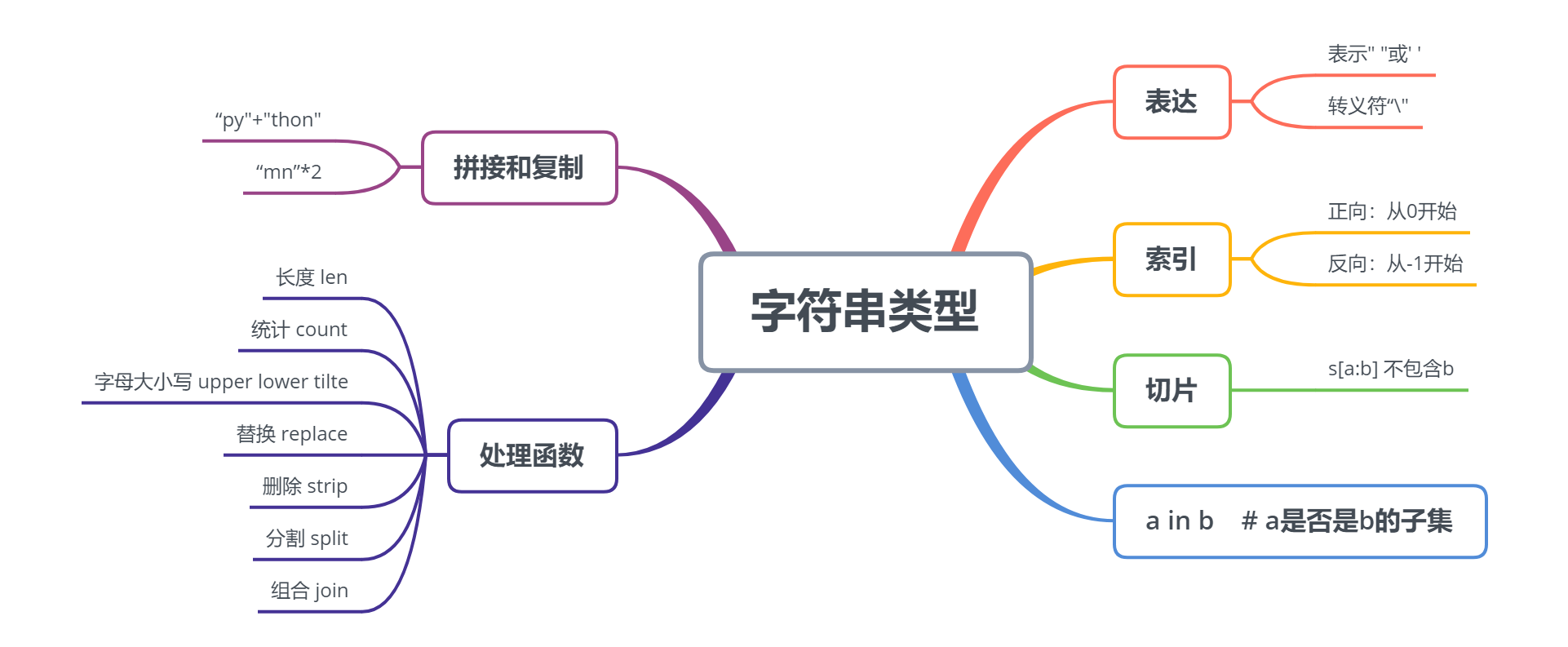## 2.1 字符串的表达

• 用""或''括起来的任意字符

print("Python")print('Python')

PythonPython

• 字符串中有双引号或单引号的情况

print("I'm 18 years old")

I'm 18 years old

print('"Python" is good')

"Python" is good

# print(""Python" is good")print("\"Python\" is good")  # \ 字符

"Python" is good

s = "py\thon"                    print(s)

python

## 2.2 字符串的性质

### 2.2.1 字符串的索引

s = "My name is Peppa Pig"

• 正向索引——从零开始递增

• 位置编号不能超过字符串的长度

• 空格也是一个位置

print(s)   print(s)print(s)

M m

s = "My name is Peppa Pig"

• 反向索引——从-1 开始递减

print(s[-1])print(s[-3])print(s[-5])

gPa

### 2.2.2 字符串的切片

• 切片间隔如不设置默认为 1，可省略

• 切片范围不包含结束位置（前闭后开）

s = "Python"print(s[0:3:1])

Pyt

print(s[0:3])

Pyt

print(s[0:3:2])

Pt

• 起始位置是 0 可以省略

• 结束位置省略，代表可以取到最后一个字符

• 可以使用反向索引

s = "Python"print(s[0:6])

Python

print(s[:6])

Python

print(s[:])

Python

print(s[-6:])

Python

• 起始位置是-1 也可以省略

• 结束位置省略，代表可以取到第一个字符

• 关键点在于-1，代表前一个位置比后一个位置大-1

s = "123456789"print(s[-1:-10:-1])

987654321

print(s[:-10:-1])

987654321

print(s[::-1])

987654321

## 2.3 字符串操作符

### 2.3.1 字符串的拼接

• 字符串 1+字符串 2

a = "I love "b = "my wife "a+b        

'I love my wife '

### 2.3.2 字符串的成倍复制

• 字符串 * n   n * 字符串

c = a+bprint(c*3)print(3*c)

I love my wife I love my wife I love my wife I love my wife I love my wife I love my wife 

### 2.2.3 成员运算

• 子集 in 全集   任何一个连续的切片都是原字符串的子集

folk_singers = "Peter, Paul and Mary"    "Peter" in folk_singers

True

"PPM" in folk_singers

False

• 遍历字符串字符   for 字符 in 字符串

for s in "Python":    print(s)

Python

## 2.4 字符串处理函数

### 2.4.1 字符串的长度

• 所含字符的个数

s = "python"len(s)

6

### 2.4.2 字符编码

• 每个单一字符对应一个唯一的互不重复的二进制编码

• Python 中使用的是 Unicode 编码

print(ord("1"))print(ord("a"))print(ord("*"))print(ord("中"))print(ord("国"))

4997422001322269

print(chr(1010))print(chr(10000))print(chr(12345))print(chr(23456))

ϲ✐〹宠

## 2.5 字符串的处理方法

### 2.5.1 字符串的分割——字符串.split(分割字符)

• 返回一个列表

• 原字符串不变

languages = "Python C C++ Java PHP R"languages_list = languages.split(" ")#括号里的参数就是我们希望对目标字符串进行分割的标记print(languages_list)print(languages)

['Python', 'C', 'C++', 'Java', 'PHP', 'R']Python C C++ Java PHP R

### 2.5.2 字符串的聚合——“聚合字符”.join(可迭代数据类型)

• 可迭代类型 如：字符串、列表

s = "12345"s_join = ",".join(s) #把可迭代的对象每一个都取出来，相邻两个之间加上聚合字符s_join

'1,2,3,4,5'

• 序列类型的元素必须是字符类型

# s = [1, 2, 3, 4, 5] 无法使用聚合s = ["1", "2", "3", "4", "5"]"*".join(s) 

'1*2*3*4*5'

### 3.5.3 删除两端特定字符——字符串.strip(删除字符)

• strip 从两侧开始搜索，遇到指定字符执行删除，遇到非指定字符，搜索停止

• 类似的还有左删除 lstrip 和右删除 rstrip

s = "      I have many blanks     "print(s.strip(" "))                        #从两端进行搜索，遇到指定字符后删除空格，然后停止print(s.lstrip(" "))print(s.rstrip(" "))print(s)

I have many blanksI have many blanks           I have many blanks      I have many blanks     

### 3.5.4 字符串的替换——字符串.replace("被替换"，"替换成")

s = "Python is coming"s1 = s.replace("Python","Py")print(s1)

Py is coming

### 3.5.5 字符串统计——字符串.count("待统计字符串")

s = "Python is an excellent language"print("an:", s.count("an"))print("e:", s.count("e"))

an: 2e: 4

### 3.3.6 字符串字母大小写

• 字符串.upper() 字母全部大写

s = "Python"s.upper()

'PYTHON'

• 字符串.lower() 字母全部小写

print(s.lower())print(s)

pythonPython

• 字符串.title()首字母大写

s.title()

'Python'

# 第三部分 布尔类型 TRUE or False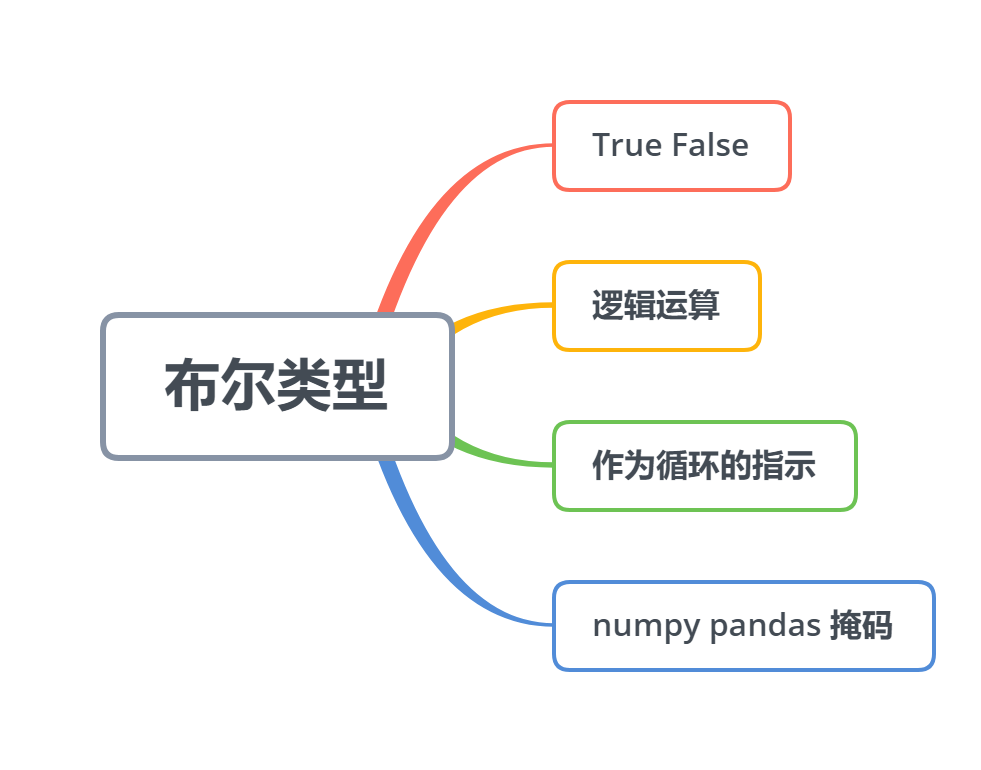## 3.1 逻辑运算的结果

a = 10print(a > 8)print(a == 12)print(a < 5)

TrueFalseFalse

• any() 数据有一个是非零就为 True

• all() 数据有一个是零就为 False （元素都是非零的）

print(any([False,1,0,None]))   # 0 False None 都是无print(all([False,1,0,None]))

TrueFalse

## 3.2 指示条件

n = 2800while True:    m = eval(input("请输入一个正整数："))    if m == n:        print("正确")        break    elif m > n:        print("太大了")    else:        print("太小了")  

请输入一个正整数：280太小了请输入一个正整数：2800正确

## 3.3 作为 numpy 数组的掩码

import numpy as npx = np.array([[1, 3, 2, 5, 7]])   # 定义 numpy数组print(x > 3)x[x > 3]

[[False False False  True  True]]array([5, 7])

# 第四部分 类型判别及类型转换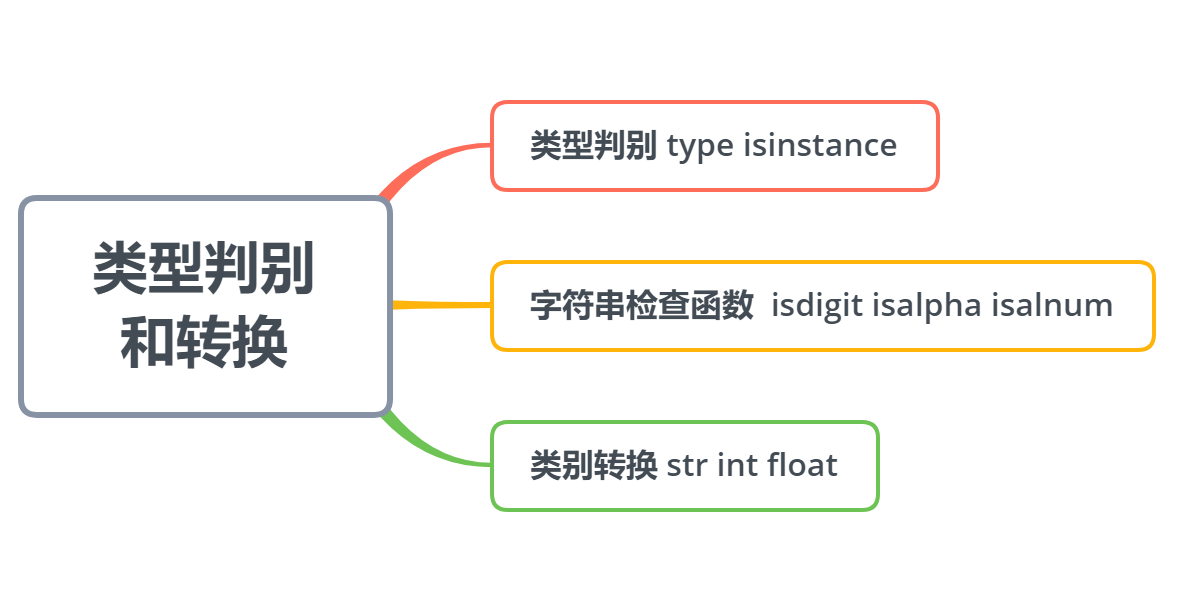## 4.1 类型判别

• type(变量)

age = 20name = "Ada"print(type(age))print(type(name))

<class 'int'><class 'str'>

• isinstance(变量,预判类型) 承认继承

• 变量类型是预判类型的子类型，则为真，否则为假

print(isinstance(age, int))        # 承认继承 这里的int就相当于是一个类

True

print(isinstance(age, object))print(isinstance(name, object))    # object 是所有类的老祖宗

TrueTrue

• 字符串检查方法

age = "20"name = "Ada"

age.isdigit()

True

name.isdigit()

False

name.isalpha()

True

age.isalpha()

False

"Ada20".isalnum()    # 比如可用于判断用户名是否合法

True

## 4.2 类型转换

• 数字类型转字符串  str(数字类型)

age = 20print("My age is "+str(age))

My age is 20

• 仅有数字组成的字符串转数字  int()  float()  eval()

s1 = "20"s2 = "10.1"

int(s1)     # 仅整型# int(s2) 会错误

20

float(s1)

20.0

float(s2)

10.1

eval(s1)

20

eval(s2)

10.1## 评论